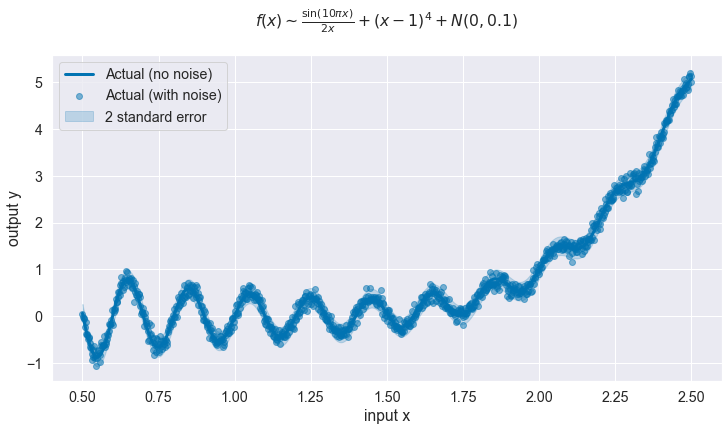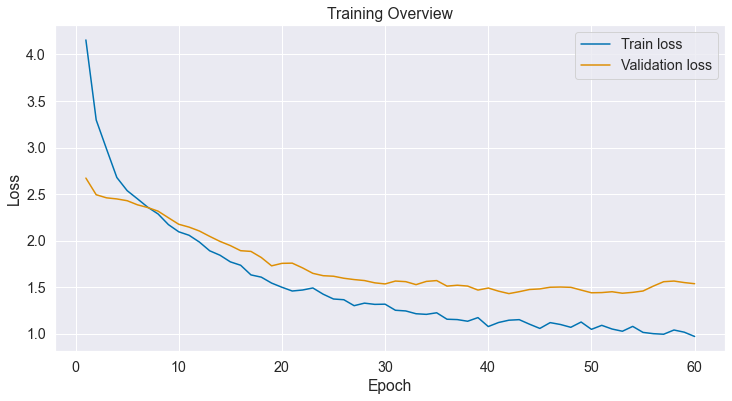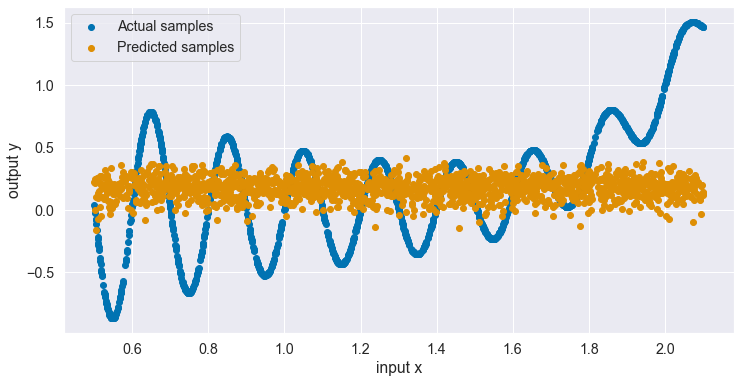# Stochastic Variational Inference (interpolation)¶

Implementation of Stochastic Variational Inference (SVI) using PyTorch, for the purpose of uncertainty quantification.

We'll consider the "Gramacy & Lee 2012" function [source]:

$f(x) = \frac{\sin(10 \pi x)}{2x} + (x-1)^4 \quad \forall x \in \mathbb{R}^+$

We'll also add a little bit of normally distributed noise around the output for good measure:

$y \sim \mathcal{N}(f(x), 0.1)$

The goal is to train a model capable of estimating its own uncertainty on unseen situations. To do so we'll split the dataset between a training / validation set on the one hand, and a test set defined on unseen input values on the other hand.

The function above is usually evaluated on the range $[0.5, 2.5]$. We'll train our model on the range $[0.5, 2.1]$ and then test it on the unseen set $[2.1, 2.5]$.

The model is expected to perform less well on the unseen set, but hopefully the decrease in performance will be flagged by an increase in uncertainty.

UPDATE: inconclusive - a single value isn't enough to predict the outcome.

## Setting up the environment¶

In :
import os

import pandas as pd
import numpy as np
import matplotlib.pyplot as plt
import seaborn as sns
import torch
import torch.nn.functional as F
from sklearn.metrics import r2_score
from sklearn.model_selection import train_test_split

cwd = os.getcwd()
if cwd.endswith('notebook'):
os.chdir('..')
cwd = os.getcwd()

In :
sns.set(palette='colorblind', font_scale=1.3)
palette = sns.color_palette()

In :
seed = 444
np.random.seed(seed);
torch.manual_seed(seed);
torch.set_default_dtype(torch.float64)


## Generating & visualising data¶

In :
def generate_samples(σ, min_x=0.5, max_x=2.5, n_samples=1000):
x = np.linspace(min_x, max_x, n_samples)[:, np.newaxis]
y = (np.sin(10*np.pi*x) / (2 * x)) + (x - 1) ** 4
dist = torch.distributions.Normal(torch.from_numpy(y), σ)
y_sample = dist.sample().detach().numpy()
return x, y, y_sample

In :
σ = 0.1
x, y, y_sample = generate_samples(σ)

In :
def plot_data(x, y, y_sample, std_err=None):
f, ax = plt.subplots(1, 1, figsize=(12, 6))

x_ = x.flatten()
y_ = y.flatten()
y_sample_ = y_sample.flatten()

ax.plot(x_, y_, '-', color=palette, linewidth=3, label='Actual (no noise)')
ax.scatter(x, y_sample_, color=palette, alpha=0.5, label='Actual (with noise)')

if std_err is not None:
ax.fill_between(x_, y_ - 2 * std_err, y_ + 2 * std_err, color=palette, alpha=0.2, label='2 standard error')

ax.set_xlabel('input x')
ax.set_ylabel('output y')
ax.set_title(r'$f(x) \sim \frac{\sin(10 \pi x)}{2x} + (x-1)^4 + N(0, 0.1)$' + '\n')
ax.legend()
return ax

In :
plot_data(x, y, y_sample, std_err=σ);## Split between different sets¶

In :
x, y, y_sample = generate_samples(σ, n_samples=10000)

In :
def compute_train_test_split(x, y, test_size):
x_train, x_test, y_train, y_test = train_test_split(x, y, test_size=test_size)
return (
torch.from_numpy(x_train),
torch.from_numpy(x_test),
torch.from_numpy(y_train),
torch.from_numpy(y_test),
)

In :
def find_cutoff_index(x, x_thres=2.1):
for i in range(len(x)):
if x[i,0] > 2.1:
return i
return i

cutoff_idx = find_cutoff_index(x)
x_train, x_val, y_train, y_val = compute_train_test_split(x[:cutoff_idx], y[:cutoff_idx], test_size=0.2)

x_test = torch.from_numpy(x[cutoff_idx:])
y_test = torch.from_numpy(y_sample[cutoff_idx:])

In :
x_train.size(), x_val.size(), x_test.size()

Out:
(torch.Size([6400, 1]), torch.Size([1600, 1]), torch.Size([2000, 1]))
In :
y_train.size(), y_val.size(), y_test.size()

Out:
(torch.Size([6400, 1]), torch.Size([1600, 1]), torch.Size([2000, 1]))
In :
class StandardScaler():
"""
Standardize data by removing the mean and scaling to unit variance.
"""
def __init__(self):
self.mean = None
self.scale = None

def fit(self, sample):
self.mean = sample.mean(0, keepdim=True)
self.scale = sample.std(0, unbiased=False, keepdim=True)
return self

def __call__(self, sample):
return self.transform(sample)

def transform(self, sample):
return (sample - self.mean) / self.scale

def inverse_transform(self, sample):
return sample * self.scale + self.mean

In :
x_scaler = StandardScaler().fit(torch.from_numpy(x))
y_scaler = StandardScaler().fit(torch.from_numpy(y_sample))


## Define model¶

In :
class VariationalModel(torch.nn.Module):
def __init__(self, n_hidden_encoder=100, n_hidden_decoder=300, n_encoding=50):
super().__init__()

self.encoder_shared1 = torch.nn.Linear(1, n_hidden_encoder)
self.encoder_shared2 = torch.nn.Linear(n_hidden_encoder, n_hidden_encoder)
self.encoder_mean = torch.nn.Linear(n_hidden_encoder, n_encoding)
self.encoder_std = torch.nn.Linear(n_hidden_encoder, n_encoding)
self.encoder_dropout = torch.nn.Dropout()

self.decoder_inner_mean = torch.nn.Linear(n_encoding, n_hidden_decoder)
self.decoder_mean = torch.nn.Linear(n_hidden_decoder, 1)
self.decoder_dropout = torch.nn.Dropout()
self.s = torch.nn.Parameter(torch.randn(()))

self.prior = torch.distributions.MultivariateNormal(
torch.zeros((n_encoding,)),
torch.Tensor([1.0] * n_encoding) * torch.eye(n_encoding),
)

def encoder(self, x):
shared = self.encoder_shared1(x)
shared = F.relu(shared)
shared = self.encoder_dropout(shared)
shared = self.encoder_shared2(shared)
shared = F.relu(shared)
shared = self.encoder_dropout(shared)

mean = self.encoder_mean(shared)
std = F.softplus(self.encoder_std(shared))

mean,
torch.diag_embed(std),
)

def decoder(self, x_enc):
decoder_mean = self.decoder_inner_mean(x_enc)
decoder_mean = F.relu(decoder_mean)
decoder_mean = self.decoder_dropout(decoder_mean)

decoder_mean = self.decoder_mean(decoder_mean)
decoder_std = F.softplus(self.s)

def forward(self, x, sample_shape=torch.Size([])):
encoder_dist = self.encoder(x)
x_enc = encoder_dist.rsample(sample_shape)
y_hat = self.decoder(x_enc)
kl_divergence = torch.distributions.kl.kl_divergence(encoder_dist, self.prior)
return y_hat, kl_divergence

In :
def compute_loss(model, x, y):
y_hat, kl_divergence = model(x)

In :
def train_one_step(model, optimizer, x_batch, y_batch):
model.train()
loss = compute_loss(model, x_batch, y_batch)
loss.backward()
optimizer.step()
return loss

def compute_rmse(model, x_test, y_test, x_scaler=None, y_scaler=None):
if x_scaler is None:
x_test_ = x_test
else:
x_test_ = x_scaler(x_test)

model.eval()
y_hat, _ = model(x_test_)
pred = y_hat.mean

if y_scaler is None:
else:
pred_ = y_scaler.inverse_transform(pred)

In :
def train(model, optimizer, scheduler, x_train_, x_val_, y_train_, y_val_, n_epochs, batch_size, print_every=10):
x_train = x_scaler(x_train_)
x_val = x_scaler(x_val_)
y_train = y_scaler(y_train_)
x_val = y_scaler(y_val_)

train_losses, val_losses = [], []
for epoch in range(n_epochs):
batch_indices = sample_batch_indices(x_train, y_train, batch_size)

batch_losses_t, batch_losses_v, batch_rmse_v = [], [], []
for batch_ix in batch_indices:
b_train_loss = train_one_step(model, optimizer, x_train[batch_ix], y_train[batch_ix])

model.eval()
b_val_loss = compute_loss(model, x_val, y_val)
b_val_rmse = compute_rmse(model, x_val, y_val)

batch_losses_t.append(b_train_loss.detach().numpy())
batch_losses_v.append(b_val_loss.detach().numpy())
batch_rmse_v.append(b_val_rmse.detach().numpy())

scheduler.step()

train_loss = np.mean(batch_losses_t)
val_loss = np.mean(batch_losses_v)
val_rmse = np.mean(batch_rmse_v)

train_losses.append(train_loss)
val_losses.append(val_loss)

if epoch == 0 or (epoch + 1) % print_every == 0:
print(f'Epoch {epoch+1} | Validation loss = {val_loss:.4f} | Validation RMSE = {val_rmse:.4f}')

_, ax = plt.subplots(1, 1, figsize=(12, 6))
ax.plot(range(1, n_epochs + 1), train_losses, label='Train loss')
ax.plot(range(1, n_epochs + 1), val_losses, label='Validation loss')
ax.set_xlabel('Epoch')
ax.set_ylabel('Loss')
ax.set_title('Training Overview')
ax.legend()

return train_losses, val_losses

def sample_batch_indices(x, y, batch_size, rs=None):
if rs is None:
rs = np.random.RandomState()

train_ix = np.arange(len(x))
rs.shuffle(train_ix)

n_batches = int(np.ceil(len(x) / batch_size))

batch_indices = []
for i in range(n_batches):
start = i + batch_size
end = start + batch_size
batch_indices.append(
train_ix[start:end].tolist()
)

return batch_indices


## Training¶

In :
learning_rate = 1e-4
momentum = 0.9
weight_decay = 5e-4
n_epochs = 60
batch_size = 128
print_every = 1

model = VariationalModel()
optimizer = torch.optim.SGD(model.parameters(), lr=learning_rate, momentum=momentum, weight_decay=weight_decay)

# Halve learning rate every X steps.
scheduler = torch.optim.lr_scheduler.LambdaLR(optimizer, lr_lambda=lambda epoch: 0.5 ** (epoch // 20))

In :
pytorch_total_params = sum(p.numel() for p in model.parameters() if p.requires_grad)
print(f'{pytorch_total_params:,} trainable parameters')

36,002 trainable parameters

In :
train(
model,
optimizer,
scheduler,
x_train,
x_val,
y_train,
y_val,
n_epochs=n_epochs,
batch_size=batch_size,
print_every=print_every,
);

Epoch 1 | Validation loss = 2.6720 | Validation RMSE = 0.5597
Epoch 2 | Validation loss = 2.4929 | Validation RMSE = 0.6748
Epoch 3 | Validation loss = 2.4598 | Validation RMSE = 0.7345
Epoch 4 | Validation loss = 2.4480 | Validation RMSE = 0.7747
Epoch 5 | Validation loss = 2.4290 | Validation RMSE = 0.7971
Epoch 6 | Validation loss = 2.3848 | Validation RMSE = 0.7934
Epoch 7 | Validation loss = 2.3553 | Validation RMSE = 0.8061
Epoch 8 | Validation loss = 2.3165 | Validation RMSE = 0.8100
Epoch 9 | Validation loss = 2.2458 | Validation RMSE = 0.7893
Epoch 10 | Validation loss = 2.1759 | Validation RMSE = 0.7738
Epoch 11 | Validation loss = 2.1453 | Validation RMSE = 0.7907
Epoch 12 | Validation loss = 2.1036 | Validation RMSE = 0.8003
Epoch 13 | Validation loss = 2.0459 | Validation RMSE = 0.7979
Epoch 14 | Validation loss = 1.9917 | Validation RMSE = 0.7980
Epoch 15 | Validation loss = 1.9468 | Validation RMSE = 0.8017
Epoch 16 | Validation loss = 1.8918 | Validation RMSE = 0.7962
Epoch 17 | Validation loss = 1.8842 | Validation RMSE = 0.8215
Epoch 18 | Validation loss = 1.8188 | Validation RMSE = 0.8082
Epoch 19 | Validation loss = 1.7292 | Validation RMSE = 0.7778
Epoch 20 | Validation loss = 1.7559 | Validation RMSE = 0.8170
Epoch 21 | Validation loss = 1.7582 | Validation RMSE = 0.8331
Epoch 22 | Validation loss = 1.7072 | Validation RMSE = 0.8126
Epoch 23 | Validation loss = 1.6486 | Validation RMSE = 0.7879
Epoch 24 | Validation loss = 1.6234 | Validation RMSE = 0.7816
Epoch 25 | Validation loss = 1.6176 | Validation RMSE = 0.7866
Epoch 26 | Validation loss = 1.5959 | Validation RMSE = 0.7818
Epoch 27 | Validation loss = 1.5817 | Validation RMSE = 0.7814
Epoch 28 | Validation loss = 1.5712 | Validation RMSE = 0.7822
Epoch 29 | Validation loss = 1.5466 | Validation RMSE = 0.7742
Epoch 30 | Validation loss = 1.5348 | Validation RMSE = 0.7728
Epoch 31 | Validation loss = 1.5657 | Validation RMSE = 0.7928
Epoch 32 | Validation loss = 1.5594 | Validation RMSE = 0.7928
Epoch 33 | Validation loss = 1.5273 | Validation RMSE = 0.7815
Epoch 34 | Validation loss = 1.5621 | Validation RMSE = 0.8010
Epoch 35 | Validation loss = 1.5709 | Validation RMSE = 0.8071
Epoch 36 | Validation loss = 1.5114 | Validation RMSE = 0.7809
Epoch 37 | Validation loss = 1.5209 | Validation RMSE = 0.7880
Epoch 38 | Validation loss = 1.5119 | Validation RMSE = 0.7862
Epoch 39 | Validation loss = 1.4690 | Validation RMSE = 0.7680
Epoch 40 | Validation loss = 1.4909 | Validation RMSE = 0.7787
Epoch 41 | Validation loss = 1.4571 | Validation RMSE = 0.7650
Epoch 42 | Validation loss = 1.4309 | Validation RMSE = 0.7531
Epoch 43 | Validation loss = 1.4515 | Validation RMSE = 0.7624
Epoch 44 | Validation loss = 1.4751 | Validation RMSE = 0.7740
Epoch 45 | Validation loss = 1.4813 | Validation RMSE = 0.7763
Epoch 46 | Validation loss = 1.4994 | Validation RMSE = 0.7847
Epoch 47 | Validation loss = 1.5018 | Validation RMSE = 0.7852
Epoch 48 | Validation loss = 1.4984 | Validation RMSE = 0.7850
Epoch 49 | Validation loss = 1.4690 | Validation RMSE = 0.7726
Epoch 50 | Validation loss = 1.4399 | Validation RMSE = 0.7605
Epoch 51 | Validation loss = 1.4420 | Validation RMSE = 0.7617
Epoch 52 | Validation loss = 1.4510 | Validation RMSE = 0.7657
Epoch 53 | Validation loss = 1.4350 | Validation RMSE = 0.7584
Epoch 54 | Validation loss = 1.4443 | Validation RMSE = 0.7619
Epoch 55 | Validation loss = 1.4590 | Validation RMSE = 0.7685
Epoch 56 | Validation loss = 1.5116 | Validation RMSE = 0.7891
Epoch 57 | Validation loss = 1.5589 | Validation RMSE = 0.8072
Epoch 58 | Validation loss = 1.5658 | Validation RMSE = 0.8092
Epoch 59 | Validation loss = 1.5495 | Validation RMSE = 0.8026
Epoch 60 | Validation loss = 1.5372 | Validation RMSE = 0.7977In :
def plot_results(x, y_sample, y_pred_sample):
f, ax = plt.subplots(1, 1, figsize=(12, 6))
ax.scatter(x.flatten(), y_sample.flatten(), color=palette, label='Actual samples')
ax.scatter(x.flatten(), y_pred_sample.flatten(), color=palette, label='Predicted samples')
ax.set_xlabel('input x')
ax.set_ylabel('output y')
ax.legend()
return ax

In :
x_val_n = x_scaler(x_val)
y_dist, _ = model(x_val_n)
y_hat_s = y_dist.mean
y_hat = y_scaler.inverse_transform(y_hat_s)

In :
val_rmse = float(compute_rmse(model, x_val, y_val, x_scaler, y_scaler).detach().numpy())
print(f'Validation RMSE = {val_rmse}')

Validation RMSE = 0.5091842581970948

In :
val_r2 = r2_score(
y_val.detach().numpy().flatten(),
y_hat.detach().numpy().flatten(),
)
print(f'Validation $R^2$ = {val_r2}')

Validation $R^2$ = -0.026665375456965235

In :
plot_results(
x_val.detach().numpy(),
y_val.detach().numpy(),
y_hat.detach().numpy(),
);In [ ]: